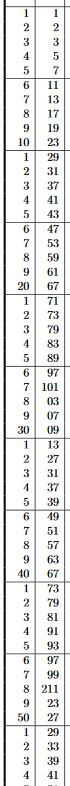Contents

# Contents

## DefinitionA prime number is a nonzero natural number that cannot be written as a product of finitely many natural numbers (all) other than itself, hence a natural number greater than 1 that is divisible only by 1 and itself.

This means that every natural number $n \in \mathbb{N}$ is, up to re-ordering of factors, uniquely expressed as a product of a tuple of prime numbers:

$n \;=\; 2^{n_1} \cdot 3^{n_2} \cdot 5^{n_3} \cdot 7^{n_4} \cdot 11^{ n_5 } \cdots$

This is called the prime factorization of $n$.

Notice that while the number $1 \in \mathbb{N}$ is, clearly, only divisible by one and by itself, hence might look like it deserves to be counted as a prime number, too, this would break the uniqueness of this prime factorization. In view of the general phenomenon in classifications in mathematics of some objects being too simple to be simple one could say that 1 is “too prime to be prime”.

However, historically, some authors did count 1 as a prime number, see e.g. Roegel 11.

## Relation to ideals and arithmetic geometry

A number is prime if and only if it generates a maximal ideal in the rig $\mathbb{N}$ of natural numbers.

Prime numbers do not quite match the prime elements of $\mathbb{N}$, since $0$ generates a prime ideal but not a maximal ideal; instead they match the irreducible elements (Wikipedia).

From the Isbell-dual point of view, where a commutative ring such as the integers $\mathbb{Z}$ is regarded as the ring of functions on some variety, namely on Spec(Z), the fact that prime numbers $p$ correspond to maximal ideals means that they correspond to the closed points in this variety (see this Example), one also writes

$(p) \in Spec(\mathbb{Z}) \,.$

This dual perspective on number theory as being the geometry (algebraic geometry) over Spec(Z) is called arithmetic geometry.

function field analogy

number fields (“function fields of curves over F1”)function fields of curves over finite fields $\mathbb{F}_q$ (arithmetic curves)Riemann surfaces/complex curves
affine and projective line
$\mathbb{Z}$ (integers)$\mathbb{F}_q[z]$ (polynomials, function algebra on affine line $\mathbb{A}^1_{\mathbb{F}_q}$)$\mathcal{O}_{\mathbb{C}}$ (holomorphic functions on complex plane)
$\mathbb{Q}$ (rational numbers)$\mathbb{F}_q(z)$ (rational functions)meromorphic functions on complex plane
$p$ (prime number/non-archimedean place)$x \in \mathbb{F}_p$$x \in \mathbb{C}$
$\infty$ (place at infinity)$\infty$
$Spec(\mathbb{Z})$ (Spec(Z))$\mathbb{A}^1_{\mathbb{F}_q}$ (affine line)complex plane
$Spec(\mathbb{Z}) \cup place_{\infty}$$\mathbb{P}_{\mathbb{F}_q}$ (projective line)Riemann sphere
$\partial_p \coloneqq \frac{(-)^p - (-)}{p}$ (Fermat quotient)$\frac{\partial}{\partial z}$ (coordinate derivation)
genus of the rational numbers = 0genus of the Riemann sphere = 0
formal neighbourhoods
$\mathbb{Z}_p$ (p-adic integers)$\mathbb{F}_q[ [ t -x ] ]$ (power series around $x$)$\mathbb{C}[ [z-x] ]$ (holomorphic functions on formal disk around $x$)
$Spf(\mathbb{Z}_p)\underset{Spec(\mathbb{Z})}{\times} X$ (“$p$-arithmetic jet space” of $X$ at $p$)formal disks in $X$
$\mathbb{Q}_p$ (p-adic numbers)$\mathbb{F}_q((z-x))$ (Laurent series around $x$)$\mathbb{C}((z-x))$ (holomorphic functions on punctured formal disk around $x$)
$\mathbb{A}_{\mathbb{Q}} = \underset{p\; place}{\prod^\prime}\mathbb{Q}_p$ (ring of adeles)$\mathbb{A}_{\mathbb{F}_q((t))}$ ( adeles of function field )$\underset{x \in \mathbb{C}}{\prod^\prime} \mathbb{C}((z-x))$ (restricted product of holomorphic functions on all punctured formal disks, finitely of which do not extend to the unpunctured disks)
$\mathbb{I}_{\mathbb{Q}} = GL_1(\mathbb{A}_{\mathbb{Q}})$ (group of ideles)$\mathbb{I}_{\mathbb{F}_q((t))}$ ( ideles of function field )$\underset{x \in \mathbb{C}}{\prod^\prime} GL_1(\mathbb{C}((z-x)))$
theta functions
Jacobi theta function
zeta functions
Riemann zeta functionGoss zeta function
branched covering curves
$K$ a number field ($\mathbb{Q} \hookrightarrow K$ a possibly ramified finite dimensional field extension)$K$ a function field of an algebraic curve $\Sigma$ over $\mathbb{F}_p$$K_\Sigma$ (sheaf of rational functions on complex curve $\Sigma$)
$\mathcal{O}_K$ (ring of integers)$\mathcal{O}_{\Sigma}$ (structure sheaf)
$Spec_{an}(\mathcal{O}_K) \to Spec(\mathbb{Z})$ (spectrum with archimedean places)$\Sigma$ (arithmetic curve)$\Sigma \to \mathbb{C}P^1$ (complex curve being branched cover of Riemann sphere)
$\frac{(-)^p - \Phi(-)}{p}$ (lift of Frobenius morphism/Lambda-ring structure)$\frac{\partial}{\partial z}$
genus of a number fieldgenus of an algebraic curvegenus of a surface
formal neighbourhoods
$v$ prime ideal in ring of integers $\mathcal{O}_K$$x \in \Sigma$$x \in \Sigma$
$K_v$ (formal completion at $v$)$\mathbb{C}((z_x))$ (function algebra on punctured formal disk around $x$)
$\mathcal{O}_{K_v}$ (ring of integers of formal completion)$\mathbb{C}[ [ z_x ] ]$ (function algebra on formal disk around $x$)
$\mathbb{A}_K$ (ring of adeles)$\prod^\prime_{x\in \Sigma} \mathbb{C}((z_x))$ (restricted product of function rings on all punctured formal disks around all points in $\Sigma$)
$\mathcal{O}$$\prod_{x\in \Sigma} \mathbb{C}[ [z_x] ]$ (function ring on all formal disks around all points in $\Sigma$)
$\mathbb{I}_K = GL_1(\mathbb{A}_K)$ (group of ideles)$\prod^\prime_{x\in \Sigma} GL_1(\mathbb{C}((z_x)))$
Galois theory
Galois group$\pi_1(\Sigma)$ fundamental group
Galois representationflat connection (“local system”) on $\Sigma$
class field theory
class field theorygeometric class field theory
Hilbert reciprocity lawArtin reciprocity lawWeil reciprocity law
$GL_1(K)\backslash GL_1(\mathbb{A}_K)$ (idele class group)
$GL_1(K)\backslash GL_1(\mathbb{A}_K)/GL_1(\mathcal{O})$$Bun_{GL_1}(\Sigma)$ (moduli stack of line bundles, by Weil uniformization theorem)
non-abelian class field theory and automorphy
number field Langlands correspondencefunction field Langlands correspondencegeometric Langlands correspondence
$GL_n(K) \backslash GL_n(\mathbb{A}_K)//GL_n(\mathcal{O})$ (constant sheaves on this stack form unramified automorphic representations)$Bun_{GL_n(\mathbb{C})}(\Sigma)$ (moduli stack of bundles on the curve $\Sigma$, by Weil uniformization theorem)
Tamagawa-Weil for number fieldsTamagawa-Weil for function fields
theta functions
Hecke theta functionfunctional determinant line bundle of Dirac operator/chiral Laplace operator on $\Sigma$
zeta functions
Dedekind zeta functionWeil zeta functionzeta function of a Riemann surface/of the Laplace operator on $\Sigma$
higher dimensional spaces
zeta functionsHasse-Weil zeta function

## Properties

### Riemann hypothesis

see at Riemann hypothesis

### Goldbach conjecture

see at Goldbach conjecture

### Asymptotic distribution

see at prime number theorem

## Specific classes of prime numbers

For historical discussion see

• Denis Roegel, A reconstruction of Lehmer’s table of primes (1914), 2011 (pdf)